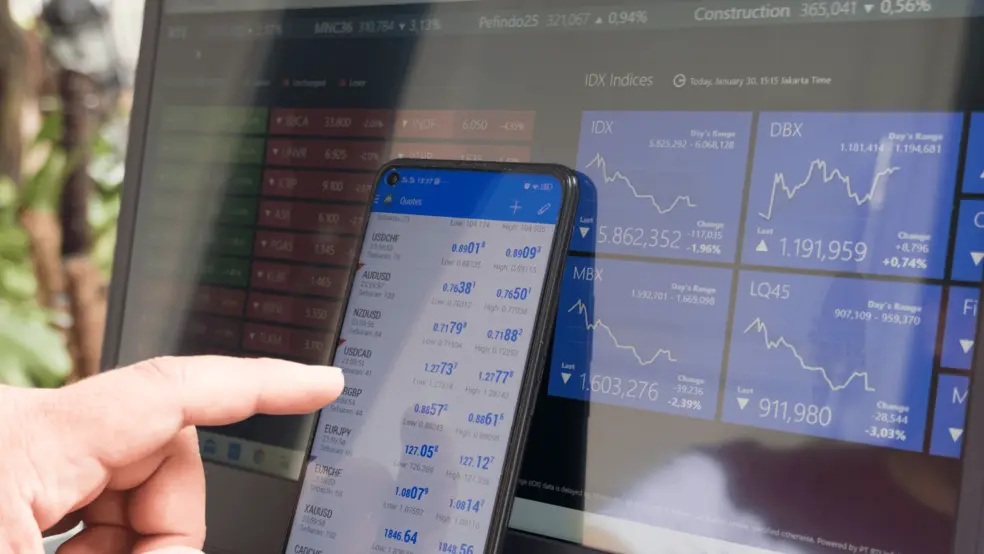Back# How to Calculate Exchange Rate Between Two Currencies

06 January 2022

We see forex rates all the time on our phones and financial news channels but, to a layman, what do they mean, how are they quoted, how are they calculated? When we say EURUSD is at 1.13, we mean, for every 100 Euros, you will get 113 U.S. dollars. Simple right? What if we say, how much Euros will you get for 100 U.S. dollars if EURUSD is 1.13? We will have to divide 100 U.S. dollars by 1.13 to get 88.50 Euros. If it's slightly confusing to convert from U.S. Dollars to Euros, it will be even more confusing to convert the two if you consider that rates are quoted in the bid and ask. Before we get too far ahead, it will be a lot simpler to understand if we know more about currency quoting convention.

#### What is the base or quote currency?

The first currency, the one on the left, in a currency pair is the base currency. The base currency is always set to the value of 1. The second currency, one on the right side, in a currency pair is the quote currency. In simple terms, the EURUSD price represents in dollars for every single Euro and, the USDEUR price represents Euros for every single Dollar.

#### What is a bid and ask?

A bid is simply the price at which a trader can sell the base currency. An ask is the price at which a trader can buy the base currency. If you consider the EURUSD quote for a bid of 1.1300 and ask of 1.1305, then it simply means that you can buy 100 Euros by selling 113.05 dollars, and you can sell 100 Euros by buying 113 dollars. If you were to buy and sell Euros at the above quote, the entire transaction would have cost you 5 cents.

Always multiple the amount with the bid or ask price, provided the currency you are converting from is the base currency. Similarly, always divide the amount with the bid-ask price, provided the currency you are converting from is the quote currency. To make it simpler, if you are converting currency from left to right (base to quote), multiply. Alternatively, if you are converting currency from right to left (base to quote), divide.

Now, it was easy to buy sell 100 Euros using the EURUSD quote but, what if we wanted to convert 100 Dollars using the EURUSD quote. As mentioned previously, we divided the 100 dollars by the quoted price but, what price should we use bid, or ask? That depends on if you are selling or buying U.S. Dollars. If you want to buy 100 U.S. dollars, then you will need to bid (sell) the Euros, hence, we use the bid rate of 1.13 (100/1.13 equals 88.50) similarly, if you want to ask (buy) the Euros we use the asking rate of 1.1305 (100/113.05 equals 88.46). Notice that if you were to buy and sell dollars at the above quote, the entire transaction would cost you 4 Euro cents.

#### How to inverse quotes

Now that we have some understanding of bid and ask and quoting convention. Let's see how we could get a USDEUR quote from EURUSD. It's simple, divide 1 from EURUSD to get USDEUR. For example, 1 divided by 1.13 equals 0.8850. Now, 0.8850 is USDEUR price. That means you get 88.50 Euros for every 100 Dollars. It is more intuitive to exchange currency when the base currency is what you want to convert. At the start, it was a bit difficult to convert 100 U.S. Dollars to Euros using the EURUSD quote but, it's much simpler using the USDEUR quote.

#### How to inverse quotes with Bid and Ask

Now that we understand how to inverse rate using one price let see how to do it using bid and ask rates. Let's get a USDEUR bid-ask quote using the above example of EURUSD bid-ask of 1.1300/1.1305. To get the USDEUR bid, we divide 1 by EURUSD ask using the above example 1/1.1305 equals 0.8845. To get the USDEUR ask price, divide 1 by EURUSD bid using the above example 1/1.1300 equals 0.8850. Thus USDEUR bid-ask is 0.8846/0.8850. Remember that the calculated bid will always be lower than your ask or something has gone wrong. Now if you were to calculate again the same 100 dollars round trip transaction it will be fairly easy to do and we will arrive at the same answer.

All the above is very important if you use, display or analyse forex data. A good understanding will stop you from making obvious errors. Also, read our blog on cross rates if you are interested in calculating exchange rates. If you have suggestions or want to ask questions feel free to contact us.